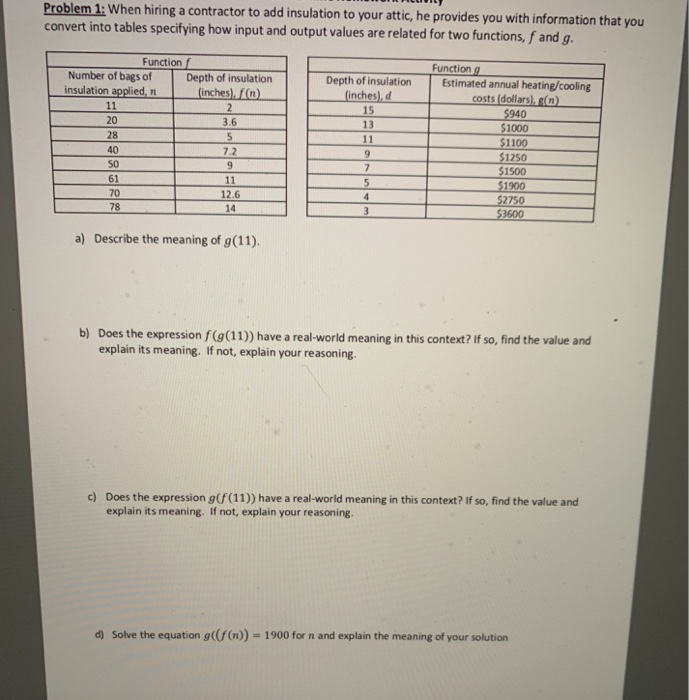# Question Solved1 AnswerProblem 1: When hiring a contractor to add insulation to your attic, he provides you with information that you convert into tables specifying how input and output values are related for two functions, f and g. Function f Function g Number of bags of 71 Depth of insulation Estimated annual heating/cooling Depth of insulation (inches) fn) 3.6 inches),d 15 13 Problem 1: When hiring a contractor to add insulation to your attic, he provides you with information that you convert into tables specifying how input and output values are related for two functions, f and g. Function f Function g Number of bags of 71 Depth of insulation Estimated annual heating/cooling Depth of insulation (inches) fn) 3.6 inches),d 15 13 20 28 40 S0 61 70 78 1000 1100 $1250$1500 7.2 12.6 14 2750 600 a) Describe the meaning of g(11). Does the expression f(g(11)) have a real-world meaning in this context? If so, find the value and explain its meaning. If not, explain your reasoning. b) c) Does the expression g(f(11)) have a real-world meaning in this context? If so, find the value and explain its meaning. If not, explain your reasoning d Solve the equation g(f(n)) 1900 for n and explain the meaning of your solutionTranscribed Image Text: Problem 1: When hiring a contractor to add insulation to your attic, he provides you with information that you convert into tables specifying how input and output values are related for two functions, f and g. Function f Function g Number of bags of 71 Depth of insulation Estimated annual heating/cooling Depth of insulation (inches) fn) 3.6 inches),d 15 13 20 28 40 S0 61 70 78 1000 1100 $1250$1500 7.2 12.6 14 2750 600 a) Describe the meaning of g(11). Does the expression f(g(11)) have a real-world meaning in this context? If so, find the value and explain its meaning. If not, explain your reasoning. b) c) Does the expression g(f(11)) have a real-world meaning in this context? If so, find the value and explain its meaning. If not, explain your reasoning d Solve the equation g(f(n)) 1900 for n and explain the meaning of your solution
More
Transcribed Image Text: Problem 1: When hiring a contractor to add insulation to your attic, he provides you with information that you convert into tables specifying how input and output values are related for two functions, f and g. Function f Function g Number of bags of 71 Depth of insulation Estimated annual heating/cooling Depth of insulation (inches) fn) 3.6 inches),d 15 13 20 28 40 S0 61 70 78 1000 1100 $1250$1500 7.2 12.6 14 2750 600 a) Describe the meaning of g(11). Does the expression f(g(11)) have a real-world meaning in this context? If so, find the value and explain its meaning. If not, explain your reasoning. b) c) Does the expression g(f(11)) have a real-world meaning in this context? If so, find the value and explain its meaning. If not, explain your reasoning d Solve the equation g(f(n)) 1900 for n and explain the meaning of your solution